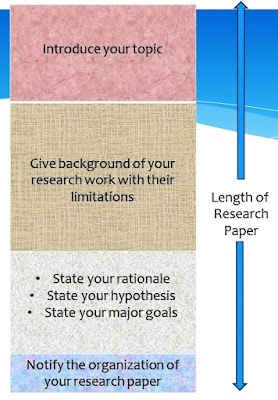How to Write an Introduction for a Research Paper? Every section of a research paper has its role and importance. Writing Introduction and Conclusion section is not an easy task. But once you understand how to write it, then it becomes very easy to write these sections. It not only requires knowledge of research work but it also requires better writing skills to attract the readers towards your paper. How? This post will explain to you...

## What is an Introduction?

• It is the second section of the research paper after the abstract section.
• It introduces the topic, summarizes the idea and context of the research work.
• It briefly explains the importance of your research work.
• It briefly explains the background literature specifically indicating their limitations and states your hypothesis indicating how your research paper overcome those limitations.
• It briefly explains the major goals of the research paper.
• Also, notify the organization of paper in the last.Fig 1. About Introduction

## When to Write an Introduction?

It is suggested by most of the researchers that the introduction section should be written just after the literature section and before the conclusion section as shown in Fig 2. Fig 2 denotes the order of writing all sections in a research paper. It is obvious to write this section in the below way because once you are aware of all literature then you can easily know the pitfall in that area. Then state your rationale for the research with major goals you will achieve that paper.Fig 2. Order of writing sections of a research paper

## Why Write an Introduction?

• To give a clear idea of your research work.
• To provide a better understanding of your research work for readers.
• To explain the requirement/ necessity of your research work.
• To explain the urgency of your research work.
• To explain the significance of your research work.

## Length of IntroductionFig 3. Length of Introduction
• The length of the introduction in the research paper depends upon the size of the research paper. If the research paper is from 5 to 7 pages of length, then the introduction can be ranging from 1 to 2 pages.
• The content size inside the introduction section is shown in Fig 3. The major content in the introduction section is of background literature. The summary of the topic and summary of research work have almost the same content size. Lastly, the organization of paper is mentioned. A standard introduction section is of 4 paragraphs. Although it depends upon the researcher's understanding.

## How to Write an Introduction?

The process of writing an introduction may vary from researcher to researcher. But an efficient introduction section must include at least four paragraphs. Each paragraph must target some important issues that a reader wants to know. Those issues are explained in the below table.
• The first paragraph introduces the research topic and research area.
• The second paragraph provides background information. It must cite the major limitations in those areas.
• The third paragraph must explain the main reason for doing that research by mentioning the importance and plan of the research. This paragraph should also include a thesis statement (hypothesis).
• The last (fourth) paragraph provides the structure of the research paper.

## KEY POINTS TO REMEMBER WHILE WRITING AN INTRODUCTION

• Explain the research area and research problem properly.
• The content of the introduction should be original and fresh. It should not be copy-paste.
• Explain all key terms/ abbreviations used in the research paper in this section only.
• The length of the introduction is important. It should be decided as per the research paper size.
• The connection between the limitation of background and proposed solution should be logically correct.
• Do not explain too much. Try to maintain the content concisely.
• The introduction should be interesting so that the new readers get engaged.
• Use simple and well-written language.
• All the content discussed in the introduction should be properly structured and well organized.
References

A Complete Guide on How to Write an Introduction for a Research PaperReviewed by IPR on May 03, 2020 Rating: 5

1.Well written sir. Nice and easily explained for beginners like me.

Regards,
2.3.4.